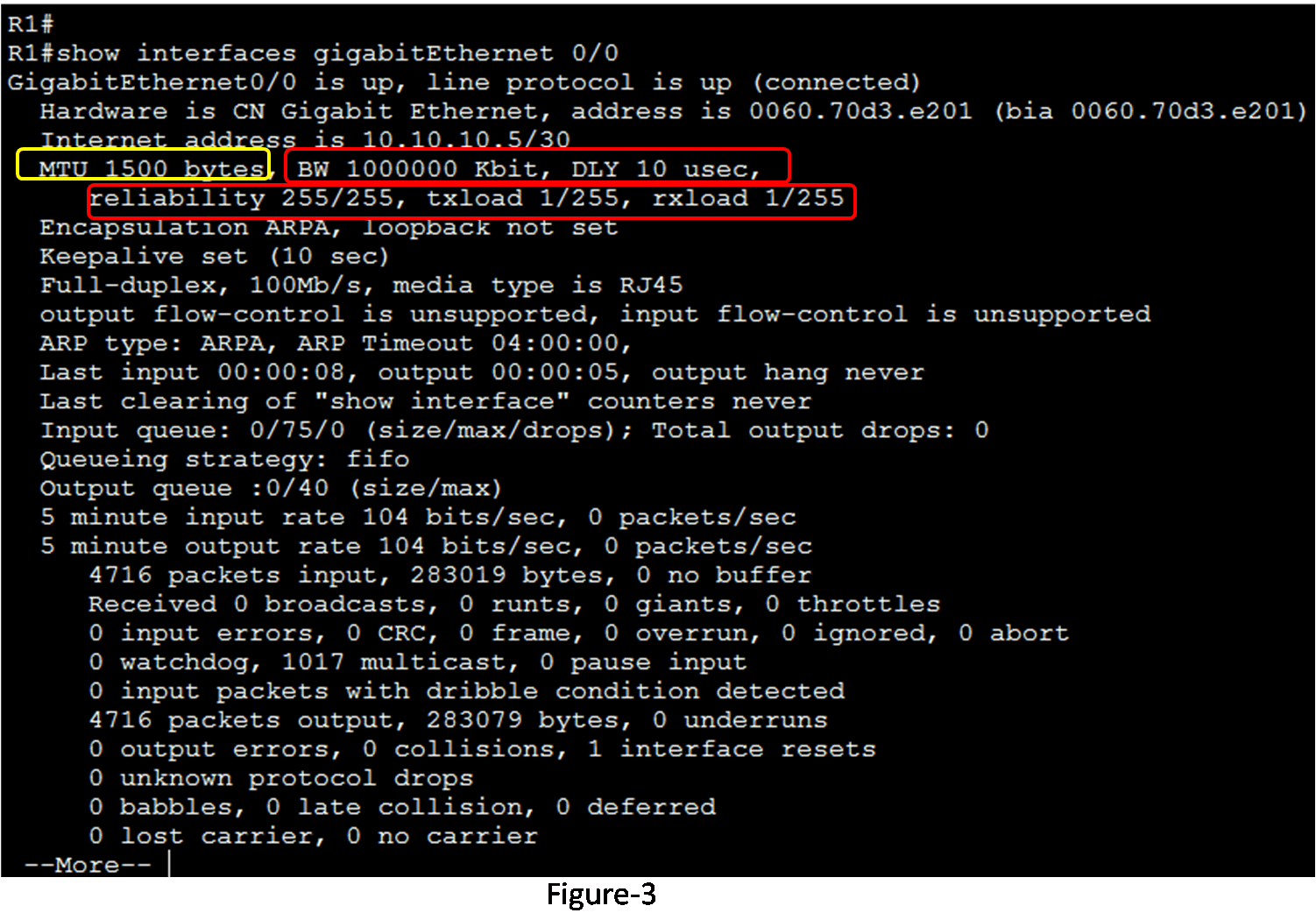# EIGRP Composite Metric

A composite metric is a number calculated based on several different components that determine the preferred route. By default, EIGRP uses bandwidth and delay to calculate the preferred path to a network. The reliability and load can also be used, but are not recommended, because they typically result in a frequent recalculation of the topology table.

• Bandwidth: The bandwidth value used in the EIGRP metric is determined by dividing 10,000,000 by the bandwidth (in kbps) of the slowest outgoing interface along the path from the source to the destination network.
• Delay: It is the cumulative value of all delays associated with all of the interfaces along the path from the source to the destination (in tens of microseconds). We can display the delay in the output of the show interfaces command.
• Reliability: It is the worst reliability between the source and destination based on keep alive.
• Load – Represents the worst load on a link between the source and destination, determined based on the packet rate and the configured bandwidth of the interface.

Figure 1 illustrates the EIGRP composite metric formula. The formula consists of K1 to K5 values, known as EIGRP metric weights. K1 bandwidth, K3 represent delay, K2 represents load, and K4 and K5 represent reliability. The bandwidth and delay values are used in the default composite metric. EIGRP for IPv4 and EIGRP for IPv6 both use the same formula for the composite metric. K1 and K3 values are set to 1 in the default formula, K2, K4 and K5 are set to 0.

##The metric calculation technique (k values) and the autonomous system number of the EIGRP must match between neighbors; otherwise, routers do not perform an adjacency. The command for changing the default k values is “metric weights” router configuration mode command. The command syntax is as under:

Router(config-router)# metric weights <tos k1 k2 k3 k4 k5>

TOS stands for the Type of Service Byte in an IPv4 packet header. It is intended to be used for a quality of service marking. But, the value of TOS must be 0. The five k values can be set into the range between 0 – 255. The metric weights value must be the same for establishing neighbor adjacencies. If metric weights values are different, then adjacency does not form.

## Verifying thekValues

We can verify the k values using the “show ip protocols” command. Figure 2 illustrates the output of this command for R1. The k values on R1 are set to the default.## Examining Interface Values

We can examine the values used in the metric calculation using the “show interfaces” command. The command also displays complete interface information. Figure 3 illustrates the show interfaces command output for the Serial 0/0/0 interface on R1. The parameters used in the metric calculation formula are marked with a red rectangle.

• BW– This is the interface bandwidth. The bandwidth is in kilobits per second.
• DLY– This is the delay of the interface. The delay is in microseconds.
• Reliability– The reliability of the interface is a fraction of 255. If the value is 255/255, it means that the reliability is 100%. The reliability is calculated as an exponential average over five minutes.
• Txload, Rxload– This is transmitted and receive load on the interface as a fraction of 255 but 255/255 is completely saturated. The value is calculated as an exponential average over five minutes.
• MTU – The MTU (Maximum Transmission Unit) is not used in the metric calculation formula. The default value of the Maximum Transmission Unit is 1500 Bytes. The Maximum Transmission Unit is carried in the EIGRP Update message to be used when tie occurred.(Visited 43 times, 43 visits today)